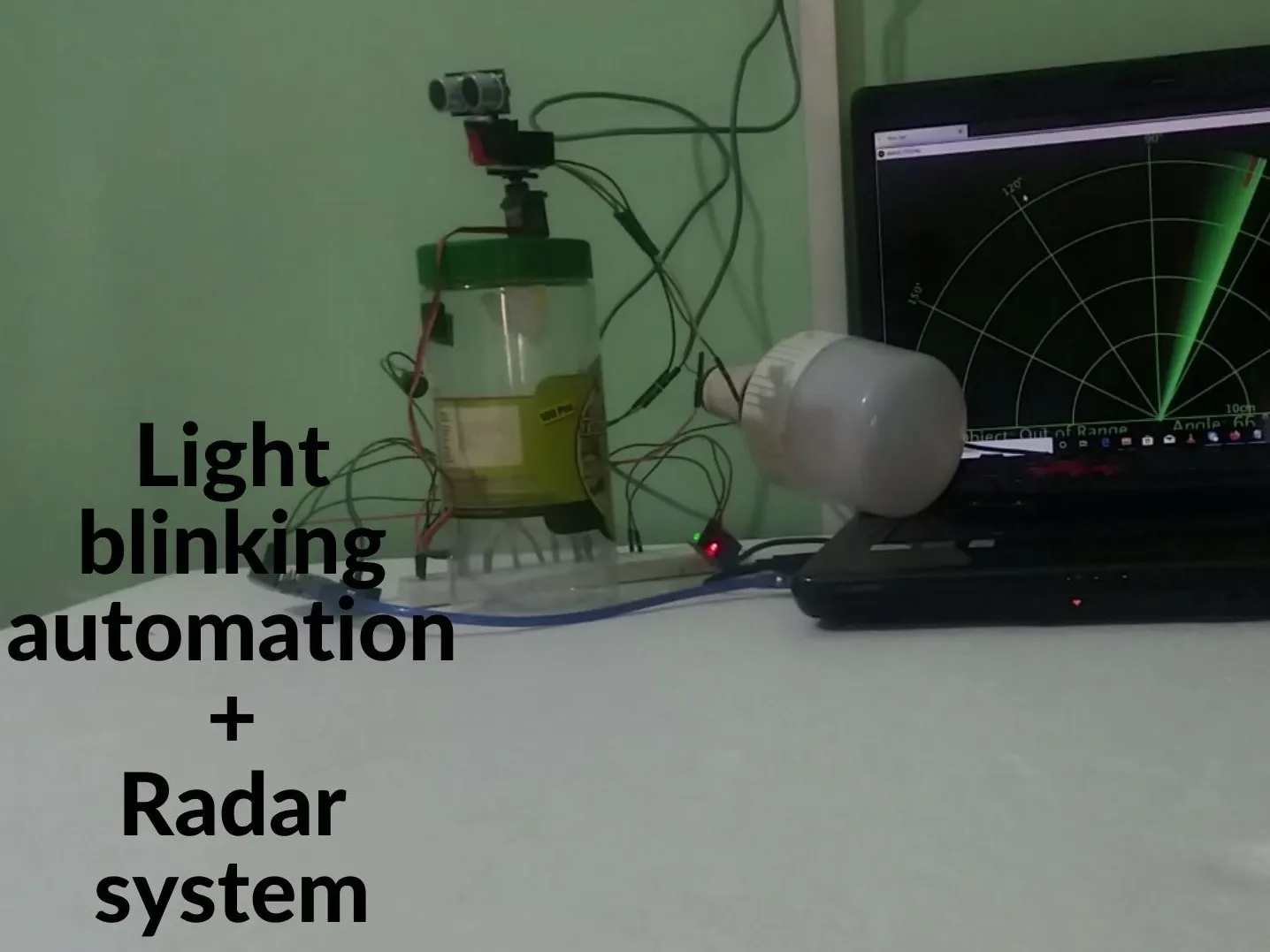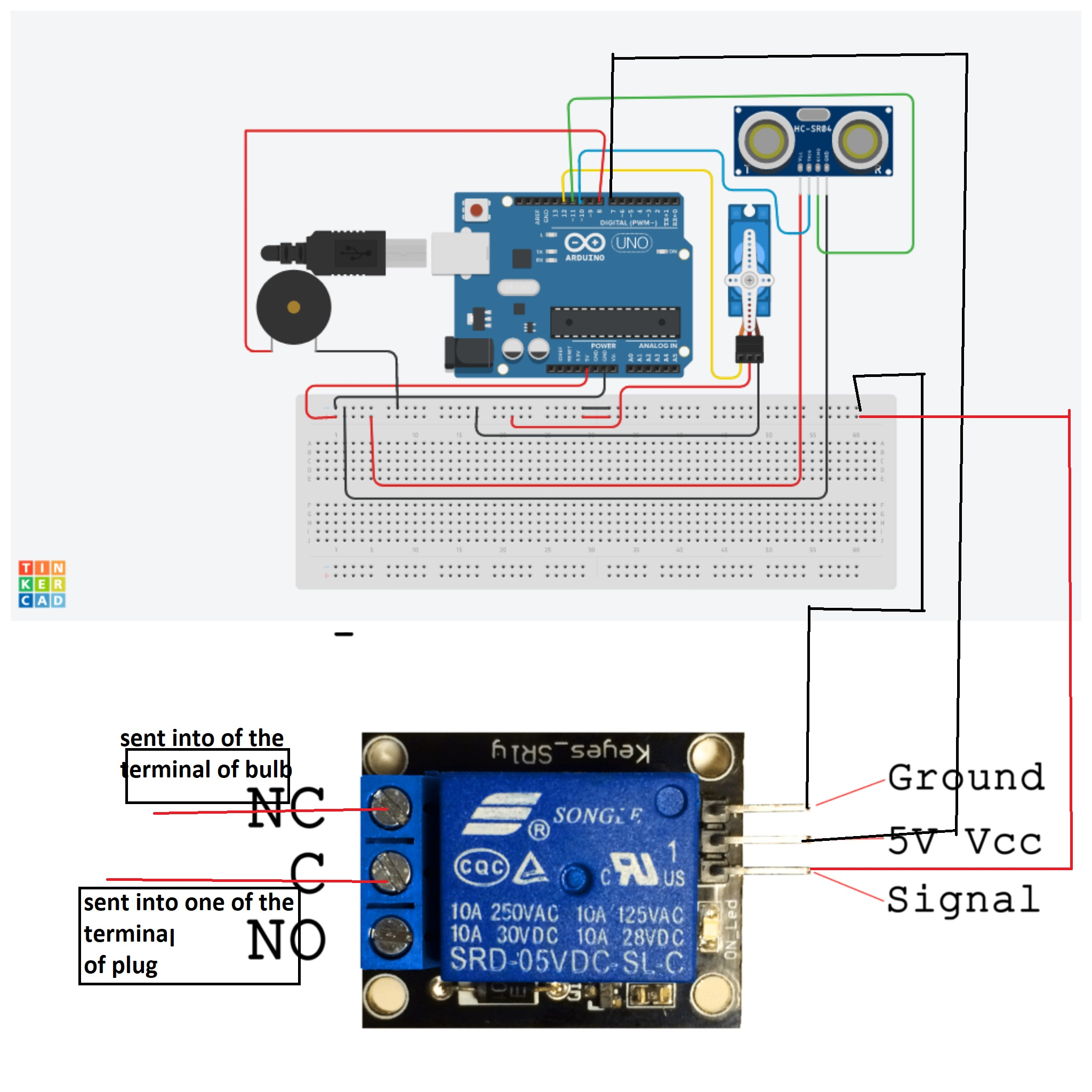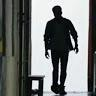when any object will find on the vision of sensor that will generate the buzzer sound and blink the led light

• 14 views
• 0 respects

## Apps and online services

In the previous tutorial, I showed you on how to make Arduino based Radar system with buzzer sound. So here I have included Ac bulb with the Radar system.

In this tutorial video I have connected one of the holder wires into N/O in Relay module. I have made mistake in that area so please connect the holder wire into N/C in Relay module .following circuit shows proper connection .

### 4.Bulb holder

Thanks UTSOURCE.net to offer electronic components for my projects

## Code

##### arduino codeC/C++
``` #include <Servo.h>
int lightRelay=7;    //pin for relay connected to light
const int trigPin = 10;
const int echoPin = 11;
const int piezoPin = 8;
// Variables for the duration and the distance
long duration;
int distance;

Servo myServo; // Creates a servo object for controlling the servo motor

void setup() {
pinMode(trigPin, OUTPUT); // Sets the trigPin as an Output
pinMode(echoPin, INPUT); // Sets the echoPin as an Input
pinMode(lightRelay,OUTPUT);//declaring relay pin of light as output
pinMode(piezoPin,OUTPUT);
Serial.begin(9600);
myServo.attach(12); // Defines on which pin is the servo motor attached
}
void loop()
{
for(int i=15;i<=165;i++){
myServo.write(i);
delay(30);
distance = calculateDistance();
if (distance <= 50 && distance >= 0) {
digitalWrite(piezoPin,HIGH);
digitalWrite(lightRelay, HIGH);
} else {
digitalWrite(piezoPin,LOW);
digitalWrite(lightRelay, LOW);
}
// Waiting 60 ms won't hurt any one
delay(60);
Serial.print(i); // Sends the current degree into the Serial Port
Serial.print(","); // Sends addition character right next to the previous value needed later in the Processing IDE for indexing
Serial.print(distance); // Sends the distance value into the Serial Port
Serial.print("."); // Sends addition character right next to the previous value needed later in the Processing IDE for indexing
}
for(int i=165;i>15;i--){
myServo.write(i);
delay(30);
distance = calculateDistance();
if (distance <= 50 && distance >= 0) {

digitalWrite(lightRelay, HIGH);
}
else {

digitalWrite(lightRelay, LOW);
}
// Waiting 60 ms won't hurt any one
delay(60);
Serial.print(i); // Sends the current degree into the Serial Port
Serial.print(","); // Sends addition character right next to the previous value needed later in the Processing IDE for indexing
Serial.print(distance); // Sends the distance value into the Serial Port
Serial.print("."); // Sends addition character right next to the previous value needed later in the Processing IDE for indexing

}
}
int calculateDistance(){
digitalWrite(trigPin, LOW);
delayMicroseconds(2);
// Sets the trigPin on HIGH state for 10 micro seconds
digitalWrite(trigPin, HIGH);
delayMicroseconds(10);
digitalWrite(trigPin, LOW);
duration = pulseIn(echoPin, HIGH); // Reads the echoPin, returns the sound wave travel time in microseconds
distance = (duration/2) / 29.1;
return distance;
}
```
##### proccesing codeC/C++
```
import processing.serial.*; // imports library for serial communication
import java.awt.event.KeyEvent; // imports library for reading the data from the serial port
import java.io.IOException;
Serial myPort; // defines Object Serial
// defubes variables
String angle="";
String distance="";
String data="";
String noObject;
float pixsDistance;
int iAngle, iDistance;
int index1=0;
int index2=0;
PFont orcFont;
void setup() {

size (1280, 720); // ***CHANGE THIS TO YOUR SCREEN RESOLUTION***
smooth();
myPort = new Serial(this,"COM5", 9600); // starts the serial communication,change this to your port type
myPort.bufferUntil('.'); // reads the data from the serial port up to the character '.'. So actually it reads this: angle,distance.

}
void draw() {

fill(98,245,31);

// simulating motion blur and slow fade of the moving line
noStroke();
fill(0,4);
rect(0, 0, width, height-height*0.065);

fill(98,245,31); // green color
// calls the functions for drawing the radar
drawLine();
drawObject();
drawText();
}
void serialEvent (Serial myPort) { // starts reading data from the Serial Port
// reads the data from the Serial Port up to the character '.' and puts it into the String variable "data".
data = data.substring(0,data.length()-1);

index1 = data.indexOf(","); // find the character ',' and puts it into the variable "index1"
angle= data.substring(0, index1); // read the data from position "0" to position of the variable index1 or thats the value of the angle the Arduino Board sent into the Serial Port
distance= data.substring(index1+1, data.length()); // read the data from position "index1" to the end of the data pr thats the value of the distance

// converts the String variables into Integer
iAngle = int(angle);
iDistance = int(distance);
}
pushMatrix();
translate(width/2,height-height*0.074); // moves the starting coordinats to new location
noFill();
strokeWeight(2);
stroke(98,245,31);
// draws the arc lines
arc(0,0,(width-width*0.0625),(width-width*0.0625),PI,TWO_PI);
arc(0,0,(width-width*0.27),(width-width*0.27),PI,TWO_PI);
arc(0,0,(width-width*0.479),(width-width*0.479),PI,TWO_PI);
arc(0,0,(width-width*0.687),(width-width*0.687),PI,TWO_PI);
// draws the angle lines
line(-width/2,0,width/2,0);
popMatrix();
}
void drawObject() {
pushMatrix();
translate(width/2,height-height*0.074); // moves the starting coordinats to new location
strokeWeight(9);
stroke(255,10,10); // red color
pixsDistance = iDistance*((height-height*0.1666)*0.025); // covers the distance from the sensor from cm to pixels
// limiting the range to 40 cms
if(iDistance<40){
// draws the object according to the angle and the distance
}
popMatrix();
}
void drawLine() {
pushMatrix();
strokeWeight(9);
stroke(30,250,60);
translate(width/2,height-height*0.074); // moves the starting coordinats to new location
popMatrix();
}
void drawText() { // draws the texts on the screen

pushMatrix();
if(iDistance>40) {
noObject = "Out of Range";
}
else {
noObject = "In Range";
}
fill(0,0,0);
noStroke();
rect(0, height-height*0.0648, width, height);
fill(98,245,31);
textSize(25);

text("10cm",width-width*0.3854,height-height*0.0833);
text("20cm",width-width*0.281,height-height*0.0833);
text("30cm",width-width*0.177,height-height*0.0833);
text("40cm",width-width*0.0729,height-height*0.0833);
textSize(40);
text("Object: " + noObject, width-width*0.875, height-height*0.0277);
text("Angle: " + iAngle +" °", width-width*0.48, height-height*0.0277);
text("Distance: ", width-width*0.26, height-height*0.0277);
if(iDistance<40) {
text("        " + iDistance +" cm", width-width*0.225, height-height*0.0277);
}
textSize(25);
fill(98,245,60);
text("30°",0,0);
resetMatrix();
text("60°",0,0);
resetMatrix();
text("90°",0,0);
resetMatrix();
text("120°",0,0);
resetMatrix();
text("150°",0,0);
popMatrix();
}
```

## Schematics